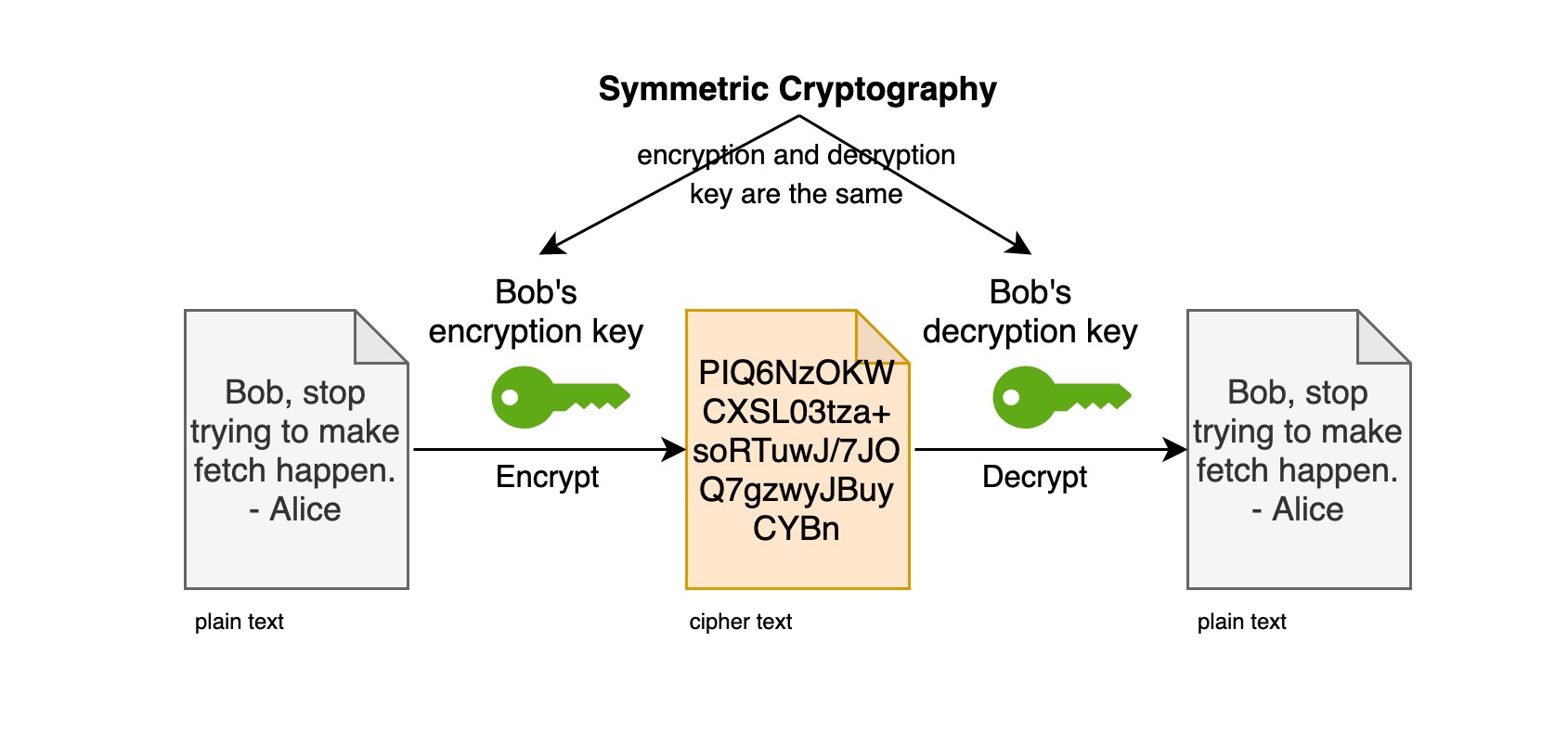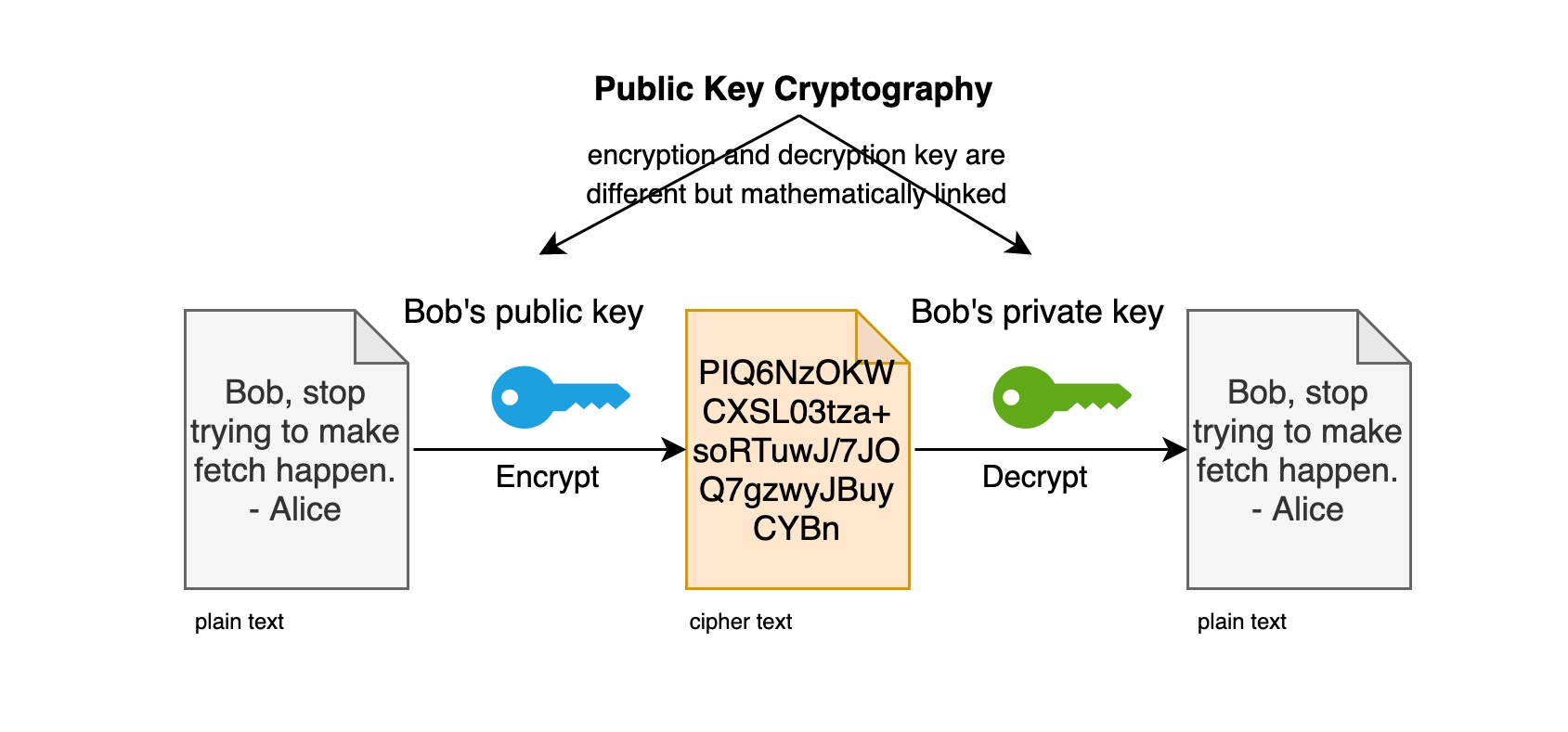## Cryptography

Cryptography is the study of the technical science of cryptography and code-breaking. The study of the objective laws of cryptographic changes, applied to the preparation of passwords to keep the secrets of communication, known as cryptography; applied to deciphering passwords to obtain communication intelligence, known as deciphering, in general, known as cryptography.

Cipher is an important means of secrecy for the communication parties to carry out special transformations of information according to the agreed laws. According to these rules, changing plaintext to ciphertext is called encryption transformation; changing ciphertext to plaintext is called decryption transformation. In the early days, ciphers only encrypted and decrypted text or digital data, but with the development of communication technology, they can encrypt and decrypt voice, image, data, etc.

## Cryptography Algorithm

What is a cryptography algorithm, usually refers to the message transformation rules used in the encryption and decryption process, is a mathematical function used to encrypt and decrypt messages. The rules used to encrypt a plaintext are called encryption algorithms, while the rules used to decrypt a ciphertext are called decryption algorithms. The operations of both encryption and decryption algorithms are usually controlled by a set of keys.

What is a key? A key (`Secret Key`) is a variable parameter in a cryptographic algorithm, usually a set of random sequences that satisfy certain conditions. The encryption key is used for the encryption algorithm and the decryption key is used for the decryption algorithm, and the encryption and decryption keys may or may not be the same.

Encryption algorithms are divided into two categories according to the key, Symmetric-key Encryption Algorithm (`Symmetric-key Encryption Algorithm`) and Asymmetric-key Encryption Algorithm (`Asymmetric-key Encryption Algorithm`).

Currently, the common single-key encryption algorithm is DES (Data Encryption Standard), and the common two-key encryption algorithm is RSA (Rivest-Shamir-Adleman), both of which were produced in the 1970s.

### Symmetric encryption

Let’s say John is a better student than Jack, and Jack wants to ask John to “help” him during the exam, but of course, they can’t say, as in our normal conversation, “Choose A for the first question, B for the second question, etc. Why? Because the invigilator understands what they are talking about, that is, this kind of communication is “explicit”, so jack thought: “need to invent a way of communication that only John and I understand”, then what did jack do? Jack went to John and said, “Look at me when I cough three times in a row, then if I touch my left ear, it means you can start to give me the answer, if there is no response, it means I am really coughing…”, then, how to pass the answer? Well, this is their “encryption algorithm”, which converts a form of the message (A, B, C, D), which we call here “plaintext”, into another form (touch the left ear, touch the right ear, put the left hand, put the right hand), here called “ciphertext”, after this conversion, it is clear that the invigilator will not understand these “ciphertext “In this way, John and jack realized the exchange of information through the form of “ciphertext”.

Symmetric encryption algorithm is also called single-key encryption, where the same set of keys is used for both encryption and decryption processes. Historically, the traditional encryption methods used by human beings are the former, such as the Enigma telegraphic code used by the German army during World War II and Morse code, which can be regarded as a single-key encryption algorithm.

Combined with the previous example, the key is a rule of “convert (A, B, C, D) into (touch left ear, touch right ear, put left hand, put right hand)”.

In fact, this set of keys for single-key encryption becomes a common secret between two or more members in order to maintain an exclusive communication link.

This sentence is well understood, right? The key is a secret shared between John and jack! Only they both know it beforehand.

So, why is it called symmetric encryption? You can understand it this way: one party encrypts the message by the key and then passes the cipher text to the other party, and the other party decrypts the cipher text by this same key and converts it into understandable plain text. The relationship between them is as follows.The following symmetric encryption algorithms are commonly used today.

 ``````1 `````` ``````DES, 3DES, AES, Blowfish, IDEA, RC5, RC6... ``````

### Asymmetric encryption

Asymmetric encryption algorithm is also known as dual-key encryption, where two sets of keys are used for the encryption and decryption process. Asymmetric encryption is a better encryption algorithm than symmetric encryption. Symmetric encryption has only one key, so the preservation of the key becomes important. Once the key is leaked, the password is also broken. Whereas in the case of asymmetric encryption, there are two keys, one is the public public key and one is the private key which is not public.

The principle of asymmetric encryption is as follows.

1. the public key and the private key are in one-to-one correspondence; there must be a public key with a corresponding, unique private key, and vice versa.
2. all (public key, private key) pairs are different.
3. the public key can be used to decrypt the information encrypted by the private key, and vice versa.
4. it should be relatively easy to generate both public and private keys, but it should be very difficult or impossible to derive the private key from the public key.

In a symmetric encryption system, the public key is used to encrypt the message and the private key is used to digitally sign it.

For example, jack wants to send a ciphertext to John, so jack starts sending a message to John as follows.

 ``````1 2 3 4 5 6 7 8 `````` ``````jack. "hi buddy, I want to send you a secret message, send me your public key." john. "No problem, here is my public key: d#8yHE8eU#hb*!neb , use this public key to encrypt your message and send it to me" jack. "Here's what I want to say to you: *&#@uehuu(**#eehu&\$##bfeu&& ``````

Why ask for the public key publicly? Here is the power of asymmetric decryption algorithm! The public key can be distributed freely, so even if it is intercepted by a third party, it is only known as the public key. If you want to decrypt the cipher text encrypted with the public key, only one person can do it, that is John, because the information encrypted by jack using John’s public key can only be decrypted by the private key corresponding to John’s public key, here is “John’s private key”! Therefore, without John’s private key, even if the third party intercepted the ciphertext, it can not be cracked! Or more strictly speaking, within a limited period of time, say thousands of years, it can not be violently cracked!

Asymmetric encryption algorithm, first of all, you need to have a pair of key pairs , one is called private key and one becomes public key; then you can distribute the public key to the user who wants to send you a cipher text, and then the user uses that public key to encrypt the cipher text, which can only be decrypted by using the private key, that is, as long as you keep your private key, you can make sure that the cipher text that others want to send to you will not be decrypted. Because of this, this kind of encryption is one-way, so it is called asymmetric encryption algorithm.This encryption algorithm is widely used, ssh/https/ssl/tls, electronic certificates, electronic signatures, electronic ID cards, etc. are all used asymmetric encryption algorithms. In fact, asymmetric encryption algorithm can be said to be the foundation of the Internet nowadays.

It should be added that asymmetric encryption is less efficient than symmetric encryption, so in the actual communication process, both asymmetric encryption and symmetric encryption are usually used to achieve data encryption transmission. The method is to first negotiate a shared secret key for symmetric encryption through asymmetric encryption, and then use the symmetric encryption secret key to encrypt the data when transferring data, in order to ensure the security while taking into account the efficiency of encrypted transmission. A similar encrypted transmission mechanism is used in https/TLS.

Because anyone can generate their own (public, private) key pairs, a reliable third-party authority is needed to generate certified (public, private) pairs in order to prevent people from spreading forged public keys to gain trust. This is the role of digital certificates, and the next article will continue to discuss what digital signatures and digital certificates are.